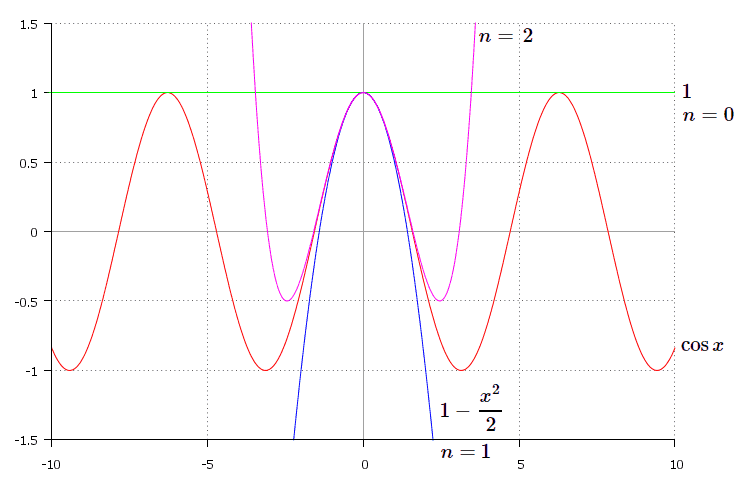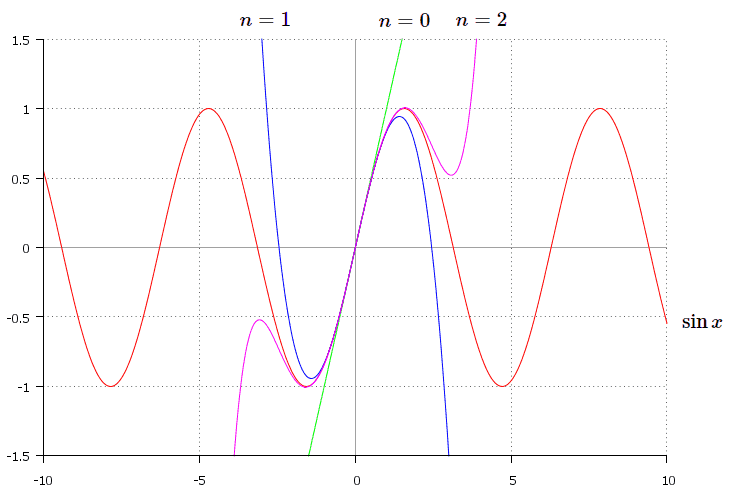# Constructing Taylor series for Sine and CosineTaylor series is used for approximation of functions. Check our article about Taylor series if you need more details. As you know, often general formula is not enough for solving problems. There’s no need in reinventing the bicycle each time, when you face one of common functions. More profitable way is to memorize a few expansions and use ready formulas when required. Previously we’ve considered Taylor expansions for exponential and logarithm (click here fore details). Let’s proceed and find formulas for sine and cosine.

## Trigonometric functions

Again, we restrict our consideration to the so called Maclaurin series. Recall that it’s Taylor series written for the vicinity of the point $x=x_0$.

Cosine function

$f(x)=\cos{x}$

At first we need derivatives. Let’s see:

$(\cos{x})^{\prime}=-\sin{x}$

$(\cos{x})^{\prime\prime}=(-\sin{x} )^{\prime}=-\cos{x}$

$(\cos{x})^{\prime\prime\prime}=(-\cos{x})^{\prime}=\sin{x}$

$(\cos{x})^{(4)}=(\sin{x})^{\prime}=\cos{x}$

So on the 4th step we get initial function and therefore further we’ll get derivatives in the same sequence.

$\cos{x} \left.\begin{matrix}\end{matrix}\right| _{x=0}=1$

Now, we need to find derivatives at the point $x_0=0$.

$(\cos{x})^{\prime}\left.\begin{matrix}\end{matrix}\right| _{x=0}=-\sin{0}=0$

$(\cos{x})^{\prime\prime}\left.\begin{matrix}\end{matrix}\right| _{x=0}=-\cos{0}=-1$

$(\cos{x})^{\prime\prime\prime}\left.\begin{matrix}\end{matrix}\right| _{x=0}=\sin{0}=0$

$(\cos{x})^{(4)}\left.\begin{matrix}\end{matrix}\right| _{x=0}=\cos{0}=1$

So we have values of derivatives in the following sequence : $0,-1,0,1$ and so on. Hence, odd powers of $x$ are absent because beside them there are zeros. Also, signs before even powers alternate beginning with $-$, then $+$, then $-$ and so on. Let’s substitute all this into general formula:

$\cos{x}=1+\frac{0}{1!} x+\frac{(-1)}{2!} x^2+\frac{0}{3!} x^3+\frac{1}{4!} x^4+⋯=\sum_{n=0}^{\infty}{\frac{(-1)^n}{(2n)!} x^{2n}}$

for $x\in R$.

On the following graph we can see the function $f(x)=\cos{x}$ and its approximations by polynomials for $n=0$, $n=1$ and $n=2$.Sine function

Now let’s consider sine function:

$f(x)=\sin{x}$

Expansion of sine into Taylor series is similar to the cosine. At first we find derivatives at the point $x_0=0$. Let’s see:

$(\sin{x})^{\prime}\left.\begin{matrix}\end{matrix}\right| _{x=0}=\cos{0}=1$

$(\sin{x})^{\prime\prime}\left.\begin{matrix}\end{matrix}\right| _{x=0}=-\sin{0}=0$

$(\sin{x})^{\prime\prime\prime}\left.\begin{matrix}\end{matrix}\right| _{x=0}=-\cos{0}=-1$

$(\sin{x})^{(4)} \left.\begin{matrix}\end{matrix}\right| _{x=0}=\sin{0}=0$

Again, on the 4th step we get initial function and therefore further we’ll get derivatives in the same sequence. Thus, we have values of derivatives in the following sequence : $1,0,-1,0$ and so on. This time even powers of $x$ are absent since zeroes are standing beside corresponding terms. Also, signs in front of the odd powers of $x$ alternate starting with $+$, then $-$, then $+$ and so on. Let’s substitute them into general formula:

$\sin{x}=0+\frac{1}{1!} x+\frac{0}{2!} x^2+\frac{(-1)}{3!} x^3+\frac{0}{4!} x^4+⋯=\sum_{n=0}^{\infty}{\frac{(-1)^n}{(2n+1)!} x^{2n+1}}$

for $x\in R$.

Here’s the graph of the function $f(x)=\sin{x}$ and its approximations by polynomials with $n=0$, $n=1$ and $n=2$.The following expansions are worth memorizing:

$e^x=1+\frac{x}{1!}+\frac{x^2}{2!}+\frac{x^3}{3!}+⋯=\sum_{n=0}^{\infty}{\frac{x^n}{n!}}, x\in R$

$\ln{(1+x)}=x-\frac{x^2}{2}+\frac{x^3}{3}-…=\sum_{n=1}^{\infty}{\frac{(-1)^{n+1} x^n}{n}}, |x|<1$

$\cos{x}=1-\frac{x^2}{2!} +\frac{x^4}{4!}+⋯=\sum_{n=0}^{\infty}{\frac{(-1)^n}{(2n)!} x^{2n}}, x\in R$

$\sin{x}= x-\frac{(x^3)}{3!}+⋯=\sum_{n=0}^{\infty}{\frac{(-1)^n}{(2n+1)!} x^{2n+1}}, x\in R$

There are two more expansions widely applied, you can obtain the formulas in similar way by yourself:

$\frac{1}{1-x}=\sum_{n=0}^{\infty}{x^n}, x\in R$

$(1+x)^{\alpha}=\sum_{n=0}^{\infty}{\frac{\alpha (\alpha-1)…(\alpha-n+1) }{n!}x^n}, |x|<1$

This is enough for solving problems, though check for more expansions of common functions if you need to.

1 Share
Filed under Math.